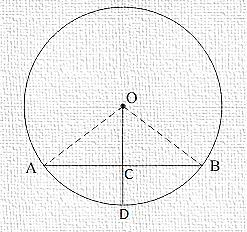Question

Radius of a circle with center O is $$41$$ units . length of a chord PQ is 80 units ,Find the distance of the chord from the  center of the circle.

Solution

Given that,Radius of circle (OA) = 41cmChord (AB) = 80cmDraw OC⊥ABWe know thatThe perpendicular from centre to chord bisects the chord∴AC=BC=802=40cmNow in ΔOCA, by Pythagoras theoremAC2 + OC2 = OA2=>402 + OC2 = 412=>1600 + OC2 = 1681=>OC2 = 81=> OC2 = 81=>OC = 81−−√=>OC = 9cmMaths

Suggest Corrections0Similar questions
View MorePeople also searched for
View More